Скачать презентацию BINOMIAL THEOREM OF NEWTON By Mayra Aydarbekova

Binomial theorem of newton.pptx

• Количество слайдов: 12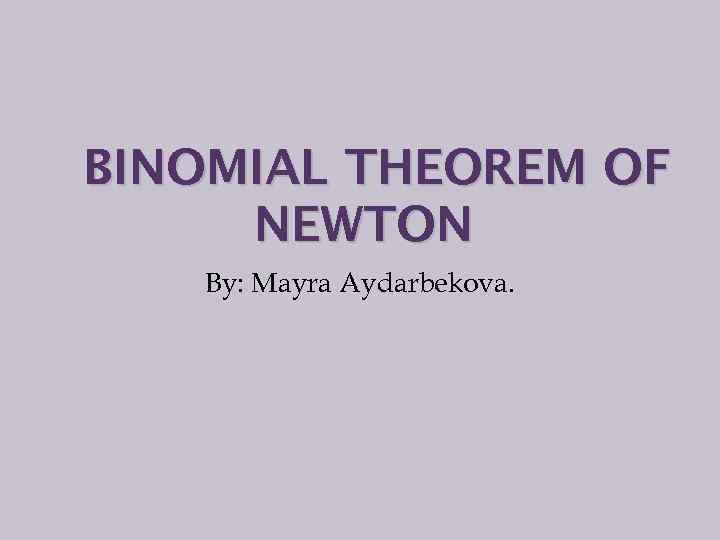BINOMIAL THEOREM OF NEWTON By: Mayra Aydarbekova.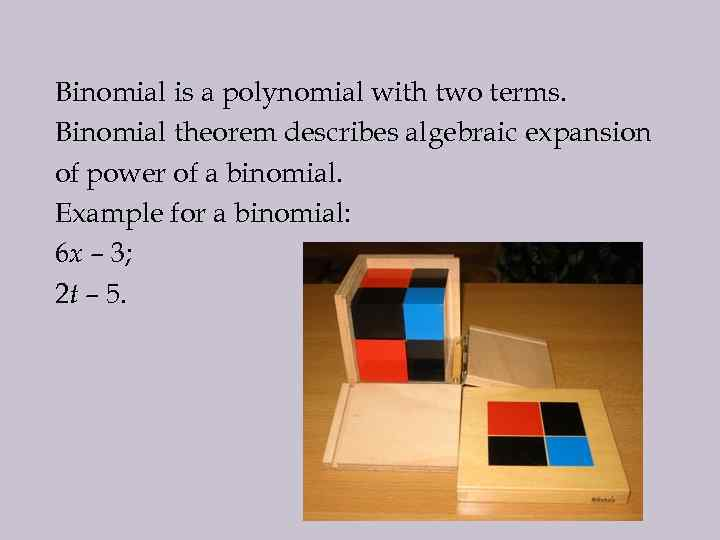Binomial is a polynomial with two terms. Binomial theorem describes algebraic expansion of power of a binomial. Example for a binomial: 6 x – 3; 2 t – 5.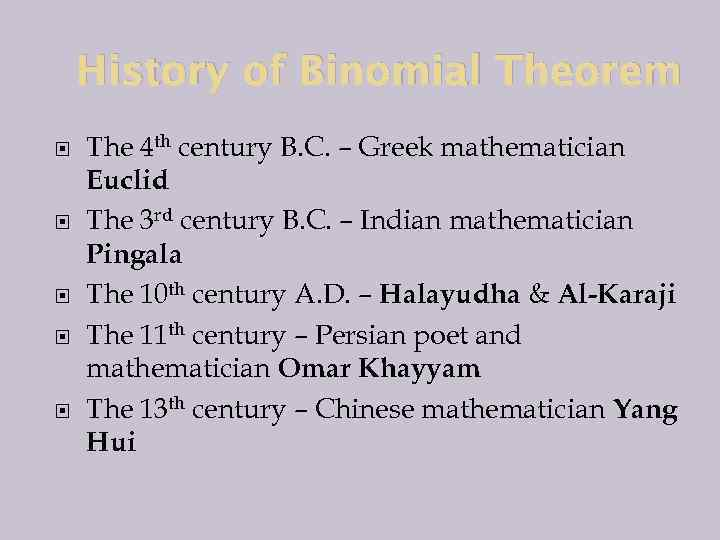History of Binomial Theorem The 4 th century B. C. – Greek mathematician Euclid The 3 rd century B. C. – Indian mathematician Pingala The 10 th century A. D. – Halayudha & Al-Karaji The 11 th century – Persian poet and mathematician Omar Khayyam The 13 th century – Chinese mathematician Yang Hui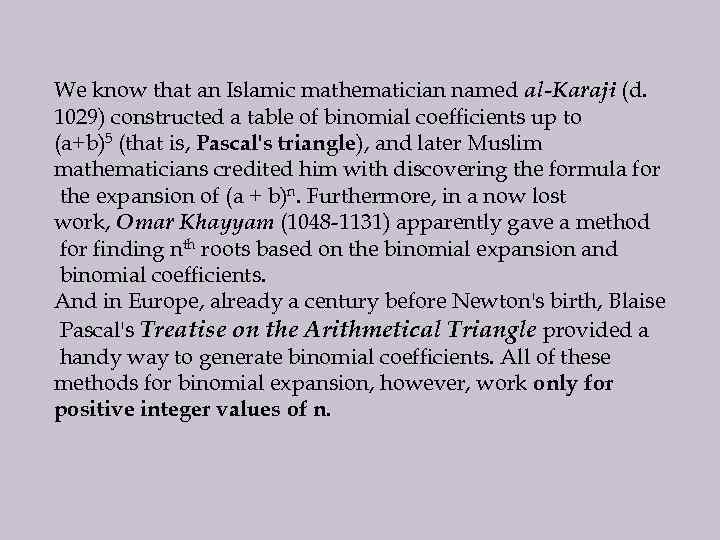We know that an Islamic mathematician named al-Karaji (d. 1029) constructed a table of binomial coefficients up to (a+b)5 (that is, Pascal's triangle), and later Muslim mathematicians credited him with discovering the formula for the expansion of (a + b)n. Furthermore, in a now lost work, Omar Khayyam (1048 -1131) apparently gave a method for finding nth roots based on the binomial expansion and binomial coefficients. And in Europe, already a century before Newton's birth, Blaise Pascal's Treatise on the Arithmetical Triangle provided a handy way to generate binomial coefficients. All of these methods for binomial expansion, however, work only for positive integer values of n.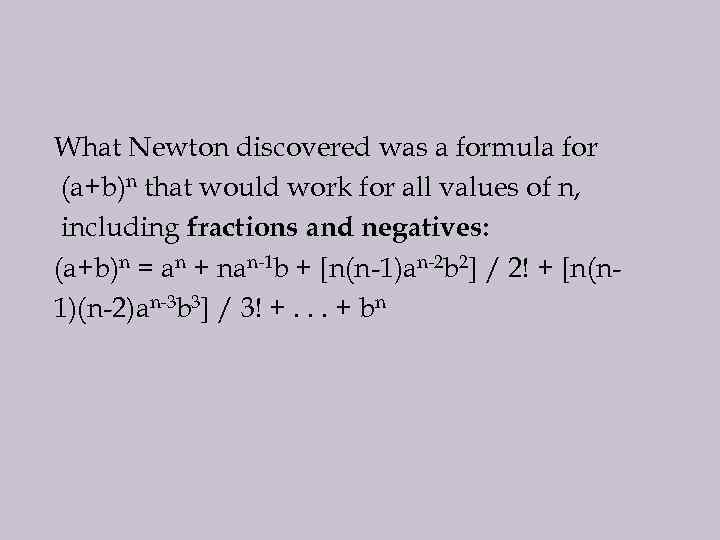What Newton discovered was a formula for (a+b)n that would work for all values of n, including fractions and negatives: (a+b)n = an + nan-1 b + [n(n-1)an-2 b 2] / 2! + [n(n 1)(n-2)an-3 b 3] / 3! +. . . + bn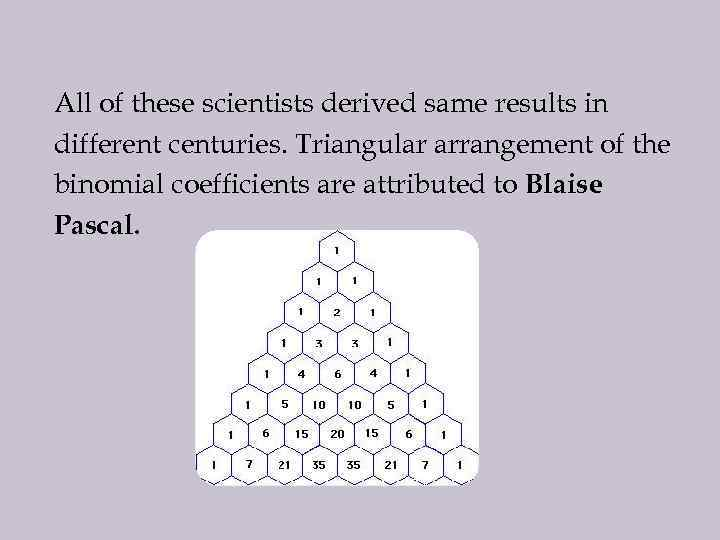All of these scientists derived same results in different centuries. Triangular arrangement of the binomial coefficients are attributed to Blaise Pascal.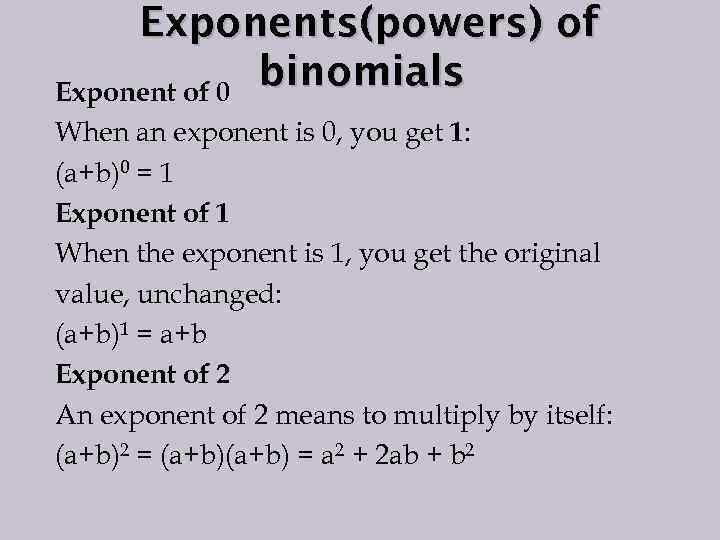Exponents(powers) of binomials Exponent of 0 When an exponent is 0, you get 1: (a+b)0 = 1 Exponent of 1 When the exponent is 1, you get the original value, unchanged: (a+b)1 = a+b Exponent of 2 An exponent of 2 means to multiply by itself: (a+b)2 = (a+b) = a 2 + 2 ab + b 2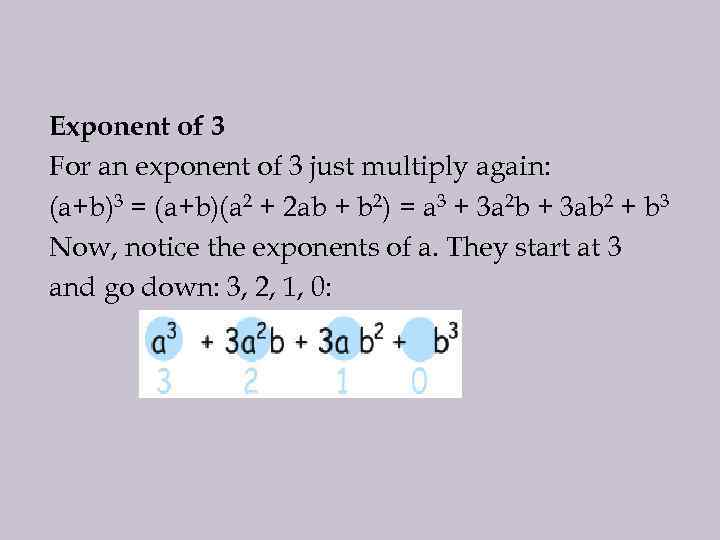Exponent of 3 For an exponent of 3 just multiply again: (a+b)3 = (a+b)(a 2 + 2 ab + b 2) = a 3 + 3 a 2 b + 3 ab 2 + b 3 Now, notice the exponents of a. They start at 3 and go down: 3, 2, 1, 0: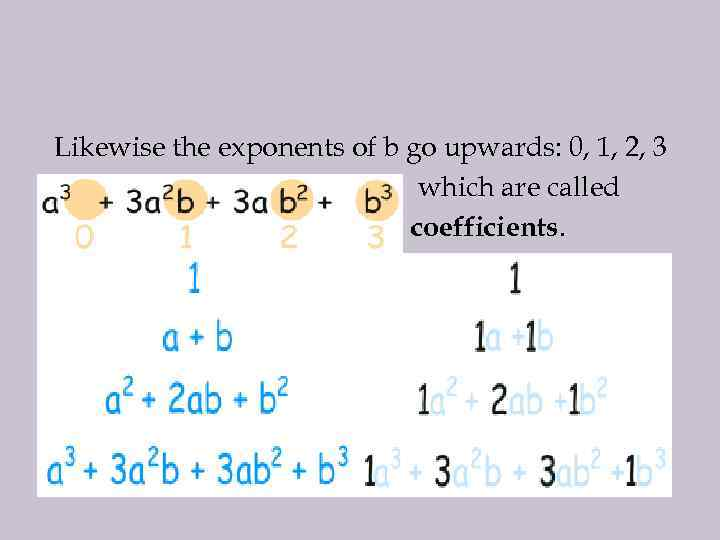Likewise the exponents of b go upwards: 0, 1, 2, 3 which are called coefficients.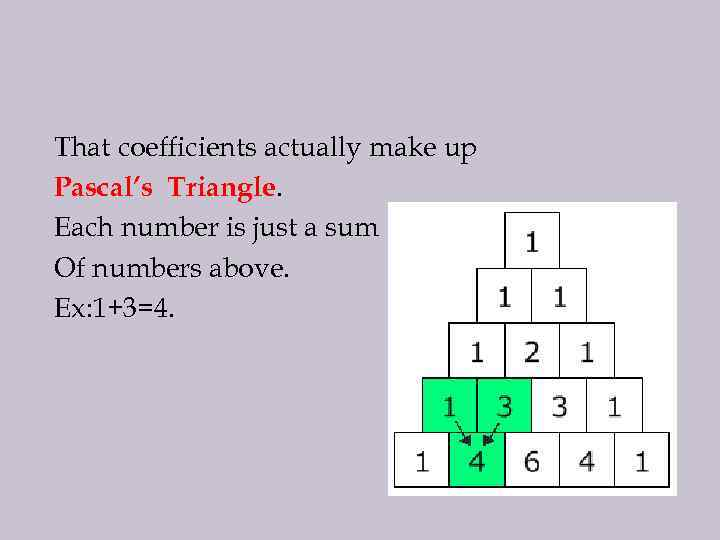That coefficients actually make up Pascal’s Triangle. Each number is just a sum Of numbers above. Ex: 1+3=4.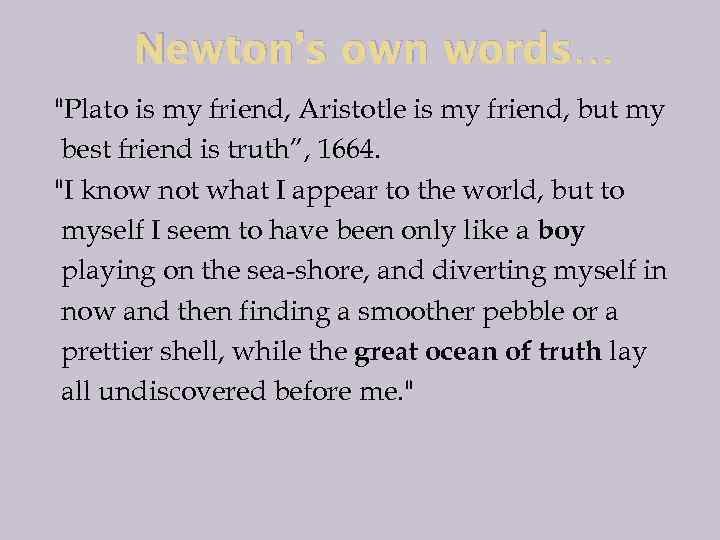Newton’s own words… "Plato is my friend, Aristotle is my friend, but my best friend is truth”, 1664. "I know not what I appear to the world, but to myself I seem to have been only like a boy playing on the sea-shore, and diverting myself in now and then finding a smoother pebble or a prettier shell, while the great ocean of truth lay all undiscovered before me. "Thanks For Attention!